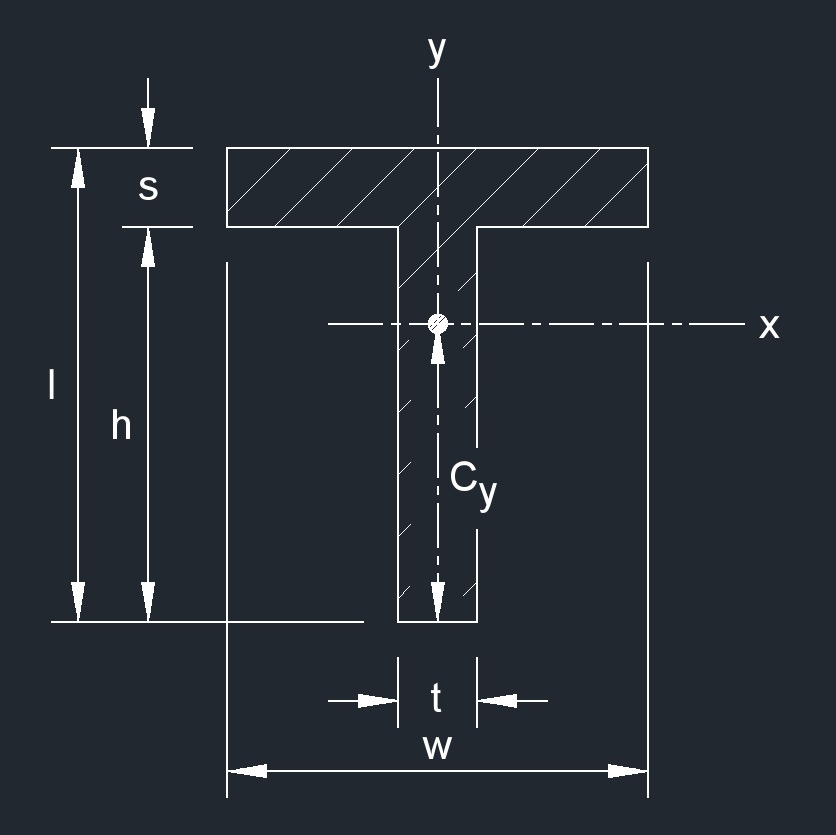# Square T Beam

Written by Jerry Ratzlaff on . Posted in Plane Geometry

•A square T beam is a structural shape used in construction.

## area of a Square T Beam formula

 $$\large{ A = w\;s + h\;t }$$

### Where:

$$\large{ A }$$ = area

$$\large{ h }$$ = height

$$\large{ t }$$ = thickness

$$\large{ s }$$ = width

$$\large{ w }$$ = width

## Distance from Centroid of a Square T Beam formulas

 $$\large{ C_x = 0 }$$ $$\large{ C_y = \frac { l^2\; t \;+\; s^2 \; \left( w \;-\; t \right) } { 2\; \left( w\;s \;+\; h\;t \right) } }$$

### Where:

$$\large{ C }$$ = distance from centroid

$$\large{ A }$$ = area

$$\large{ h }$$ = height

$$\large{ l }$$ = height

$$\large{ t }$$ = thickness

$$\large{ s }$$ = width

$$\large{ w }$$ = width

## Elastic section Modulus of a Square T Beam formulas

 $$\large{ S_{x} = \frac { I_{x} }{ C_{y} } }$$ $$\large{ S_{y} = \frac { I_{y} }{ C_{x} } }$$

### Where:

$$\large{ S }$$ = elastic section modulus

$$\large{ C }$$ = distance from centroid

$$\large{ I }$$ = moment of inertia

## Perimeter of a Square T Beam formula

 $$\large{ P = 2\; \left( w + h + s \right) }$$

### Where:

$$\large{ P }$$ = perimeter

$$\large{ h }$$ = height

$$\large{ s }$$ = width

$$\large{ w }$$ = width

## Polar Moment of Inertia of a Square T Beam formulas

 $$\large{ J_{z} = I_{x} + I_{y}{^2} }$$ $$\large{ J_{z1} = I_{x1} + I_{y1}{^2} }$$

### Where:

$$\large{ J }$$ = torsional constant

$$\large{ I }$$ = moment of inertia

## Radius of Gyration of a Square T Beam formulas

 $$\large{ k_{x} = \sqrt { \frac{ I_{x} }{ A } } }$$ $$\large{ k_{y} = \sqrt { \frac{ I_{y} }{ A } } }$$ $$\large{ k_{z} = \sqrt { k_{x}{^2} + k_{y}{^2} } }$$ $$\large{ k_{x1} = \sqrt { \frac{ I_{x1} }{ A } } }$$ $$\large{ k_{y1} = \sqrt { \frac{ I_{y1} }{ A } } }$$ $$\large{ k_{z1} = \sqrt { k_{x1}{^2} + k_{y1}{^2} } }$$

### Where:

$$\large{ k }$$ = radius of gyration

$$\large{ A }$$ = area

$$\large{ I }$$ = moment of inertia

## Second Moment of Area of a Square T Beam formulas

 $$\large{ I_{x} = \frac{ t\;C_{y}{^3} \;+\; w \; \left( l \;-\; C_y \right)^3 \;-\; \left( w \;-\; t \right) \; \left( l \;-\; C_y \;-\; s \right)^3 }{3} }$$ $$\large{ I_{x} = \frac{ h\;t^3 }{12} + \frac{ w^3 \;s }{12} }$$ $$\large{ I_{x1} = I_{x} + A \;C_{y} }$$ $$\large{ I_{y1} = I_{y} + A\; C_{x} }$$

### Where:

$$\large{ I }$$ = moment of inertia

$$\large{ A }$$ = area

$$\large{ C }$$ = distance from centroid

$$\large{ h }$$ = height

$$\large{ t }$$ = thickness

$$\large{ s }$$ = width

$$\large{ w }$$ = width

## Torsional Constant of a Square T Beam formula

 $$\large{ J = \frac{ w\;s^3 \;+\; l \;-\; \left( \frac {s}{2} \right) \; t^3 }{3} }$$

### Where:

$$\large{ J }$$ = torsional constant

$$\large{ l }$$ = height

$$\large{ t }$$ = thickness

$$\large{ s }$$ = width

$$\large{ w }$$ = width